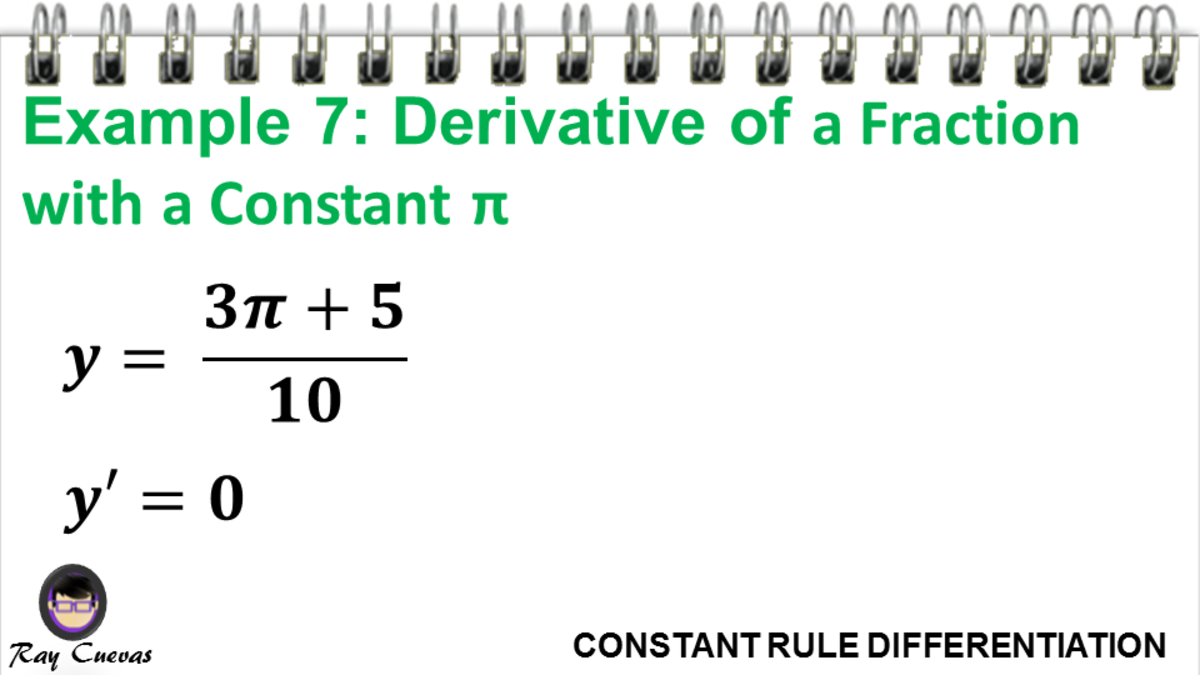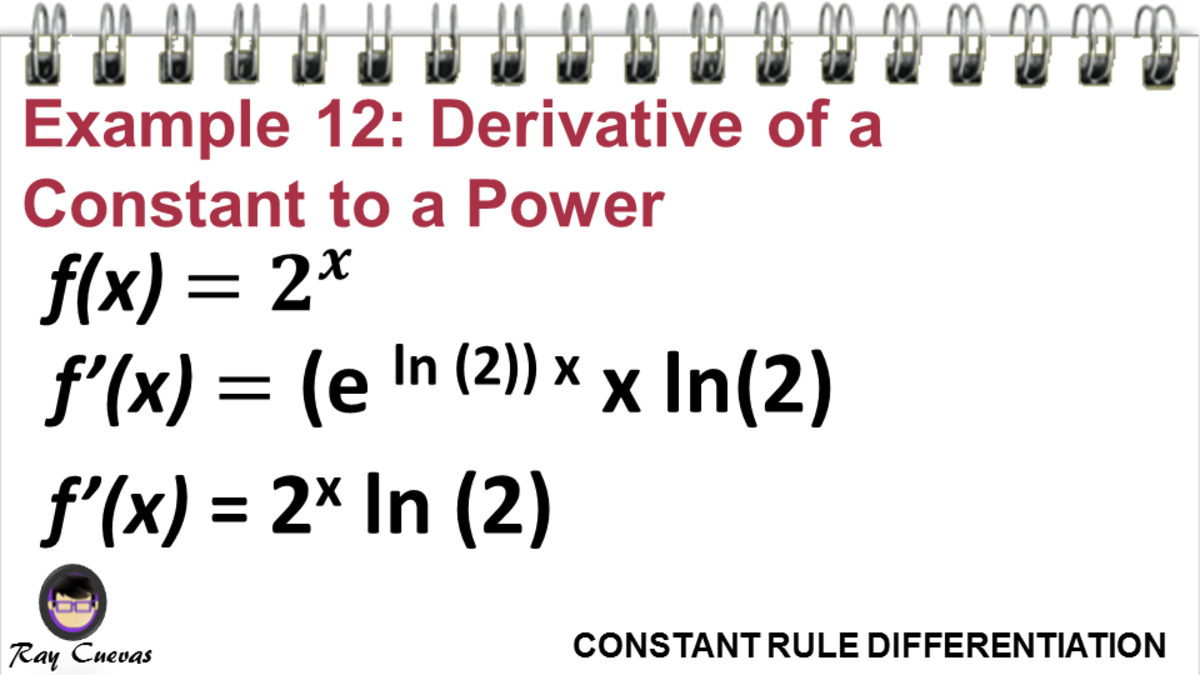# The Derivative of a Constant (With Examples)

• Author:
• Updated date:

Ray is a licensed engineer in the Philippines. He loves to write about mathematics and civil engineering.

## What is the Derivative of a Constant?

The derivative of a constant is always zero. The Constant Rule states that if f(x) = c, then f’(c) = 0 considering c is a constant. In Leibniz notation, we write this differentiation rule as follows:

d/dx (c) = 0

A constant function is a function, whereas its y does not change for variable x. In layman's terms, constant functions are functions that do not move. They are principally numbers. Consider constants as having a variable raised to the power zero. For instance, a constant number 5 can be 5x0, and its derivative is still zero.

The derivative of a constant function is one of the most basic and most straightforward differentiation rules that students must know. It is a rule of differentiation derived from the power rule that serves as a shortcut to finding the derivative of any constant function and bypassing solving limits. The rule for differentiating constant functions and equations is called the Constant Rule.

The Constant Rule is a differentiation rule that deals with constant functions or equations, even if it is a π, Euler's number, square root functions, and more. In graphing a constant function, the result is a horizontal line. A horizontal line imposes a constant slope, which means there is no rate of change and slope. It suggests that for any given point of a constant function, the slope is always zero.

## Why Is the Derivative of a Constant Zero?

Ever wondered why the derivative of a constant is 0?

We know that dy/dx is a derivative function, and it also means that the values of y are changing for the values of x. Hence, y is dependent on the values of x. Derivative means the limit of the change ratio in a function to the corresponding change in its independent variable as the last change approaches zero.

A constant remains constant irrespective of any change to any variable in the function. A constant is always a constant, and it is independent of any other values existing in a particular equation.

The derivative of a constant comes from the definition of a derivative.

f′(x) = lim h→0 [f(x+h)−f(x)] / h

f′(x) = lim h→0 (c−c) /h

f′(x) = lim h→0 0

f′(x) = 0

Scroll to Continue

To further illustrate that the derivative of a constant is zero, let us plot the constant on the y-axis of our graph. It will be a straight horizontal line as the constant value does not change with the change in the value of x on the x-axis. The graph of a constant function f(x) = c is the horizontal line y=c which has slope = 0. So, the first derivative f' (x) is equal to 0.

## Example 1: Derivative of a Constant Equation

What is the derivative of y = 4?

The first derivative of y = 4 is y’ = 0.

## Example 2: Derivative of a Constant Equation F(X)

Find the derivative of the constant function f(x) = 10.

The first derivative of the constant function f(x) = 10 is f’(x) = 0.

## Example 3: Derivative of a Constant Function T(X)

What is the derivative of the constant function t(x) = 1?

The first derivative of the constant function t(x) = 1 is t’(x) = 1.

## Example 4: Derivative of a Constant Function G(X)

Find the derivative of the constant function g(x) = 999.

The first derivative of the constant function g(x) = 999 is still g’(x) = 0.

## Example 5: Derivative of Zero

Find the derivative of 0.

The derivative of 0 is always 0. This example still falls under the derivative of a constant.

## Example 6: Derivative of Pi

What is the derivative of π?

The value of π is 3.14159. Still a constant, so the derivative of π is zero.

## Example 7: Derivative of a Fraction with a Constant Pi

Find the derivative of the function (3π + 5) / 10.

The given function is a complex constant function. Therefore, its first derivative is still 0.Example 7: Derivative of a Fraction with a Constant PiJohn Ray Cuevas

## Example 8: Derivative of the Euler's Number "e"

What is the derivative of the function √(10) / (e−1)?

The exponential "e" is a numerical constant that is equal to 2.71828. Technically, the function given is still constant. Hence, the first derivative of the constant function is zero.

## Example 9: Derivative of a Fraction

What is the derivative of the fraction 4 / 8?

The derivative of 4 / 8 is 0.

## Example 10: Derivative of a Negative Constant

What is the derivative of the function f(x) = -1099?

The derivative of the function f(x) = -1099 is 0.

## Example 11: Derivative of a Constant to a Power

Find the derivative of ex.

Note that e is a constant and has a numerical value. The given function is a constant function raised to the power of x. According to the derivative rules, the derivative of ex is the same as its function. The slope of the function ex is constant, wherein that for every x-value, the slope is equal to every y-value. Therefore, the derivative of ex is 0.Example 11: Derivative of a Constant to a PowerJohn Ray Cuevas

## Example 12: Derivative of a Constant Raised to the X Power

What is the derivative of 2x?

Rewrite 2 to a format that contains an Euler number e.

2x = (e ln (2))x ln(2)

2x = 2x ln (2)

Therefore, the derivative of 2x is 2x ln(2).Example 12: Derivative of a Constant Raised to the X PowerJohn Ray Cuevas

## Example 13: Derivative of a Square Root Function

Find the derivative of y = √81.

The given equation is a square root function √81. Remember that a square root is a number multiplied by it to get the resulting number. In this case, √81 is 9. The resulting number 9 is called the square of a square root.

Following the Constant Rule, the derivative of an integer is zero. Therefore, f' (√81) is equal to 0.

## Example 14: Derivative of a Trigonometric Function

Extract the derivative of the trigonometric equation y = sin (75°).

The trigonometric equation sin (75°) is a form of sin (x) where x is any degree or radian angle measure. If to get the numerical value of sin (75°), the resulting value is 0.969. Given that sin (75°) is 0.969. Therefore, its derivative is zero.

## Example 15: Derivative of a Summation

Given the summation ∑x=110 (x2)

The given summation has a numerical value, which is 385. Thus, the given summation equation is a constant. Since it is a constant, y' = 0.